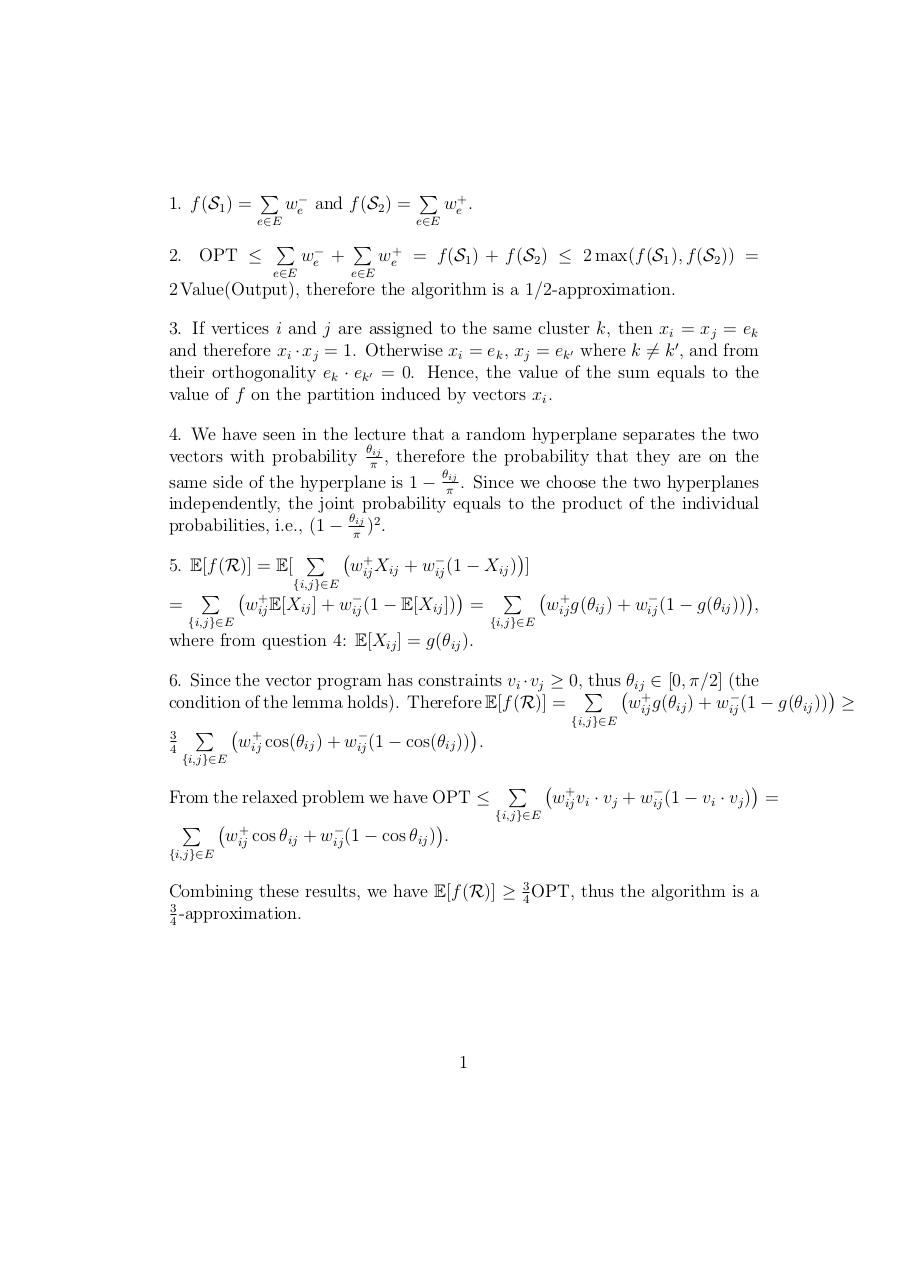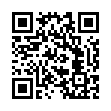# correlation .pdf

### File information

Original filename: correlation.pdf

This PDF 1.5 document has been generated by TeX / pdfTeX-1.40.14, and has been sent on pdf-archive.com on 25/02/2016 at 11:34, from IP address 195.111.x.x. The current document download page has been viewed 392 times.
File size: 87 KB (1 page).
Privacy: public file

## Download original PDF file

correlation.pdf (PDF, 87 KB)

### Document preview

1. f (S1 ) =

P

we− and f (S2 ) =

2. OPT ≤

e∈E

we+ .

e∈E

e∈E

P

P

we− +

P

we+ = f (S1 ) + f (S2 ) ≤ 2 max(f (S1 ), f (S2 )) =

e∈E

2 Value(Output), therefore the algorithm is a 1/2-approximation.
3. If vertices i and j are assigned to the same cluster k, then xi = xj = ek
and therefore xi · xj = 1. Otherwise xi = ek , xj = ek0 where k 6= k 0 , and from
their orthogonality ek · ek0 = 0. Hence, the value of the sum equals to the
value of f on the partition induced by vectors xi .
4. We have seen in the lecture that a random hyperplane separates the two
θ
vectors with probability πij , therefore the probability that they are on the
θ
same side of the hyperplane is 1 − πij . Since we choose the two hyperplanes
independently, the joint probability equals to the product of the individual
θ
probabilities, i.e., (1 − πij )2 .

P
+

5. E[f (R)] = E[
wij
Xij + wij
(1 − Xij ) ]
{i,j}∈E

P
P
+

+

=
wij E[Xij ] + wij
(1 − E[Xij ]) =
wij
g(θij ) + wij
(1 − g(θij )) ,
{i,j}∈E

{i,j}∈E

where from question 4: E[Xij ] = g(θij ).
6. Since the vector program has constraints vi ·vj ≥ 0, P
thus θij ∈ [0, π/2] (the

+

condition of the lemma holds). Therefore E[f (R)] =
wij
g(θij ) + wij
(1 − g(θij )) ≥
{i,j}∈E

P
+

3
w
cos(θ
)
+
w
(1

cos(θ
))
.
ij
ij
ij
ij
4
{i,j}∈E

P
+

From the relaxed problem we have OPT ≤
wij
vi · vj + wij
(1 − vi · vj ) =
{i,j}∈E

P
+

wij cos θij + wij (1 − cos θij ) .
{i,j}∈E

Combining these results, we have E[f (R)] ≥ 34 OPT, thus the algorithm is a
3
-approximation.
4

1### Link to this page

#### Permanent link

Use the permanent link to the download page to share your document on Facebook, Twitter, LinkedIn, or directly with a contact by e-Mail, Messenger, Whatsapp, Line..

#### Short link

Use the short link to share your document on Twitter or by text message (SMS)

#### HTML Code

Copy the following HTML code to share your document on a Website or Blog

#### QR Code### Related keywords